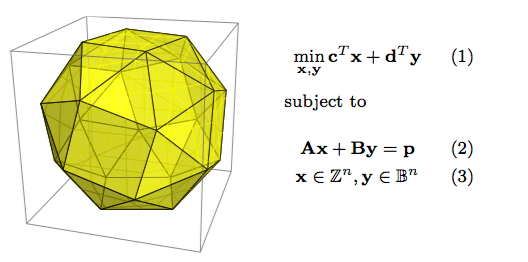# Combinatorial Optimization (B4M35KO+BE4M35KO)

## Exam results

• 23-05-2022: password is the animal at the “Theodor taking a walk” instance from HW2 (czech, lower case)

## Course overviewThis course is focusing on the problems and algorithms of combinatorial optimization (often called discrete optimization). Following the courses on linear algebra, graph theory, and basics of optimization, we show optimization techniques based on graphs, integer linear programming, heuristics, approximation algorithms and state space search methods. We focus on application of optimization, e.g. logistics, transportation, planning of human resources, scheduling in production lines, message routing and scheduling in parallel computers.

## Prerequisities

Optimization, Discrete Mathematics, Logics and Graph Theory.

## Lectures

This timetable is for the czech version (B4M35KO) of the course. The timetable of the english version (BE4M35KO) is provided by prof. Hanzálek.

Week No. Dates Title Notes Handouts Stream
1 14.2.-20.2. Introduction of Basic Terms, Example Applications https://rtime.felk.cvut.cz/~hanzalek/KO/Basic_e.pdf Intro
2 21.2.-27.2. Integer Linear Programming - Algorithms https://rtime.felk.cvut.cz/~hanzalek/KO/ILP_e.pdf ILP-I
3 28.2.-6.3. Integer Linear Programming - Problem Formulations https://rtime.felk.cvut.cz/~hanzalek/KO/ILP_e.pdf ILP-II
4 7.3.-13.3. Shortest Paths https://rtime.felk.cvut.cz/~hanzalek/KO/SPT_e.pdf SP-I
SP-II
SP-III
5 14.3.-20.3. Problem Formulation by Shortest Paths Theory test 1. https://rtime.felk.cvut.cz/~hanzalek/KO/SPT_e.pdf
6 21.3.-27.3. Flows and Cuts - Algorithms and Problem Formulation https://rtime.felk.cvut.cz/~hanzalek/KO/Flows_e.pdf Flows-I
Flows-II
Flows-III
7 28.3.-3.4. Bipartite Matching. Multi-commodity Network Flows https://rtime.felk.cvut.cz/~hanzalek/KO/Flows_e.pdf
8 4.4.-10.4. Knapsack Problem, Pseudo-polynomial and Approximation Algorithms https://rtime.felk.cvut.cz/~hanzalek/KO/knapsack_e.pdf Knapsack-I
9 11.4.-17.4. Traveling Salesman Problem and Approximation Algorithms https://rtime.felk.cvut.cz/~hanzalek/KO/TSP_e.pdf TSP-I
10 18.4.-24.4. Mono-processor Scheduling Theory test 2. https://rtime.felk.cvut.cz/~hanzalek/KO/sched_e.pdf Sched-I
Sched-II
Sched-III
Sched--IV
11 25.4.-1.5. Scheduling on Parallel Processors https://rtime.felk.cvut.cz/~hanzalek/KO/sched_e.pdf
12 2.5.-8.5. Project Scheduling with Time Windows https://rtime.felk.cvut.cz/~hanzalek/KO/sched_e.pdf
13 9.5.-15.5. Constraint Programming https://rtime.felk.cvut.cz/~hanzalek/KO/cp_e.pdf CP-I
14 16.5.-22.5. Reserve

Materials by topic

Students may receive a total of 100 points. The final exam is worth 50% of the grade, while the other 50% can be obtained during the semester.

The points are distributed according to the following table.

 Category Maximum points Semester Homeworks (4 assignments) 17 Theoretical tests (two tests, 8 points each) 16 Practical test 8 Semester project 9 Minimum points Total semester 1) 50 30 Final exam 2) Written part 40 20 Oral exam 10 0 Total 100 50

1) To get an ungraded assessment the following requirements have to be met:

• successfully solve all homework assignments (i.e., accepted by BRUTE evaluation as correct)
• obtain at least 30 from 50 points

The last date of awarding the ungraded assessments is 22. 5. 2022. If you fail to fulfill the requirements by then, you will also fail the entire class.

2) Only the students, who obtained the ungraded assessment are allowed to take the exam. The exam constitutes of the written part (40 points) and oral exam (10 points). To pass the written exam, it is necessary to get at least 20 points. The oral exam is mandatory.

Points [0,50) [50,60) [60,70) [70,80) [80,90) [90,100]
Grade F E D C B A

### Assessment recognition

The following applies only to students who got an ungraded assessment in the previous year (all other students have to undergo the course from scratch).

Although the assessments are not fully recognized, the students may opt-in to transfer some points from the previous year to the current one (they may also undergo the course from scratch to get a higher number of points). The following rules apply

1. Theoretical tests, practical test and the homeworks have to be repeated.
2. Students may transfer points from the semester project. If the grading table of the course changes between the years, the points are scaled accordingly. It is also possible to work on the same topic to increase/decrease the number of received points.
3. The minimum number of points required to get an ungraded assessment is always taken from the current year grading table.